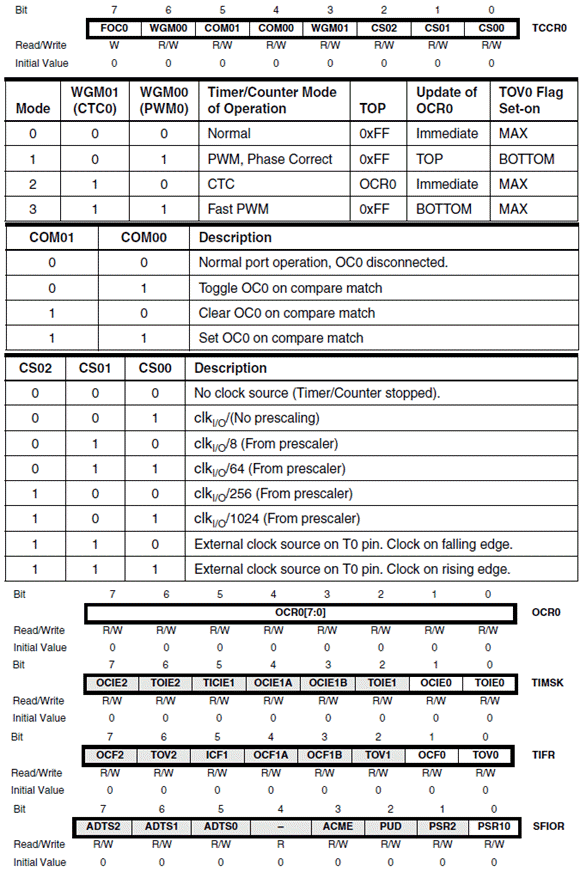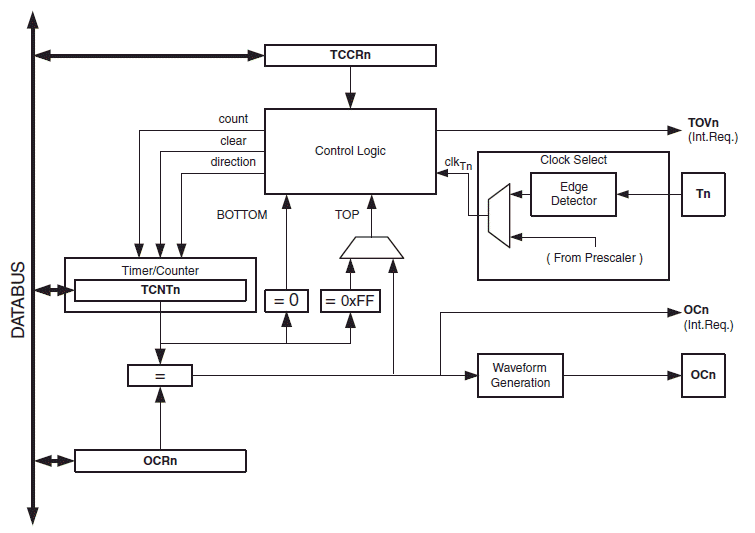# AVR Timer Calculator

## Normal Mode

Inputs:
Calculate Count value from CPU freq. and pre-scalar:

Results:Equations Used:
$$C=256-\frac{F_{CPU}}{N F_{d}}$$
where, N is the pre-scalar value
$$F_d=\frac{1}{T_d}$$
where $$T_d$$ is the required time delay
The timer CPU period is, $$T_{CPU}=N\times(\frac{1}{F_{CPU}})$$
and the number of CPU clocks required for the specified time delay is, $$N_c=\frac{T_d}{T_{CPU}}$$

### About Normal Mode Calculator/Time Delay Calculator

The above online Normal Mode calculator helps you to calculate the timer/counter 0 count value(C) that is to be loaded into the TCNT0 register for given CPU frequency(oscillator frequency, FCPU) and required time delay(Td). It also calculates the CPU time period for given CPU frequency and the number of clocks or ticks that is required for specified time delay. This calculator can be used for making time delay routine for ATmega32, ATMega328p and Arduino. This is illustrated in the tutorial Time delay using timers without inbuild functions in Arduino.

The following are links to worked out examples with normal mode online calculator.## CTC Mode

Inputs:
OCR0, Timer/Counter0 frequency & delay Calculator in CTC mode

Results:Equations Used:
$$C=\frac{F_{osc}}{2 N F_{w}}-1$$
where, Fosc is the frequency of the CPU, Fw is the output square wave frequency and N is the pre-scalar value.

The above online CTC Mode calculator helps you to calculate the timer/counter 0 count value(C) that is to be loaded into the OCR0 register(Output Compare Register 0) for given CPU frequency(or oscillator frequency, Fosc) and desired output wave frequency(Fw).The following are links to worked out examples with online CTC mode calculator.
- Programming ATmega328p in CTC mode
- Arduino CTC mode Programming with Examples

## Fast PWM Mode

### Non-Inverted Fast PWM Mode

Caculates OCR0, Wave Frequency & Wave Time Period
Inputs:

%

Results:

### Inverted Mode Fast PWM Mode:

Calculates OCR0, Wave Frequency & Wave Time Period
Inputs:

%

Results:Equations Used:
$$F_{w(non-inv)}=\frac{F_{osc}}{256N},$$ $$OCR0_{non-inv} = \frac{256D}{100} - 1,$$ $$F_{w(inv)}=\frac{F_{osc}}{256N},$$ $$OCR0_{inv} = 255 - \frac{256D}{100}$$

The above online Fast PWM calculator calculates the wave frequency and count value to be loaded into OCR0 register for non-inverted and inverted mode.The following are links to worked out examples for Fast PWM mode:
- ATmega328P Fast PWM mode Programming Examples - Programming Arduino Timer 0 in Fast PWM mode - Programming Arduino Timer 1 in Fast PWM mode - Programming Arduino Timer 2 in Fast PWM mode

## Phase Correct PWM Mode

### Non-Inverted Phase Correct PWM Mode

Caculates OCR0, Wave Frequency & Wave Time Period Inputs:

%

Results:

### Inverted Mode Fast PWM Mode

Calculates OCR0, Wave Frequency & Wave Time Period
Inputs:

%

Results:Equations Used:
$$F_{w(non-inv)}=\frac{Fosc}{510N},$$ $$OCR0_{non-inv}= \frac{255D}{100},$$ $$F_{w(non-inv)}=\frac{Fosc}{510N},$$ $$OCR0_{non-inv}= 255 - \frac{255D}{100}$$

### About Phase Correct PWM Calculator

The above online Phase Correct PWM calculator calculates the wave frequency and count value to be loaded into OCR0 register for non-inverted and inverted mode. The followings are links to worked out examples with phase correct PWM online calculator:

- Phase Correct PWM with ATmega328P - Programming Timer Counter in Phase Correct PWM mode with ATmega32